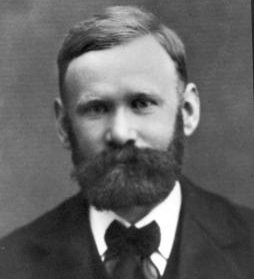Agner Krarup Erlang (1878 – 1929) Danish mathematician, statistician and engineer, who invented queueing theory and the Gamma law.

This tiny post is about some basics on the Gamma law, one of my favorite laws. Recall that the Gamma law ${\mathrm{Gamma}(a,\lambda)}$ with shape parameter ${a\in(0,\infty)}$ and scale parameter ${\lambda\in(0,\infty)}$ is the probability measure on ${[0,\infty)}$ with density

$x\in[0,\infty)\mapsto\frac{\lambda^a}{\Gamma(a)}x^{a-1}\mathrm{e}^{-\lambda x}$

where we used the eponymous Euler Gamma function

$\Gamma(a)=\int_0^\infty x^{a-1}\mathrm{e}^{-x}\mathrm{d}x.$

Recall that ${\Gamma(n)=(n-1)!}$ for all ${n\in\{1,2,\ldots\}}$. The normalization ${\lambda^a/\Gamma(a)}$ is easily recovered from the definition of ${\Gamma}$ and the fact that if ${f}$ is a univariate density then the scaled density ${\lambda f(\lambda\cdot)}$ is also a density for all ${\lambda>0}$, hence the name “scale parameter” for ${\lambda}$. So what we have to keep in mind essentially is the Euler Gamma function, which is fundamental in mathematics far beyond probability theory.

The Gamma law has an additive behavior on its shape parameter under convolution, in the sense that for all ${\lambda\in(0,\infty)}$ and all ${a,b\in(0,\infty)}$,

$\mathrm{Gamma}(a,\lambda)*\mathrm{Gamma}(b,\lambda)=\mathrm{Gamma}(a+b,\lambda).$

The Gamma law with integer shape parameter, known as the Erlang law, is linked with the exponential law since ${\mathrm{Gamma}(1,\lambda)=\mathrm{Expo}(\lambda)}$ and for all ${n\in\{1,2,\ldots\}}$

$\mathrm{Gamma}(n,\lambda) =\underbrace{\mathrm{Gamma}(1,\lambda)*\cdots*\mathrm{Gamma}(1,\lambda)}_{n\text{ times}} =\mathrm{Expo}(\lambda)^{*n}.$

In queueing theory and telecommunication modeling, the Erlang law ${\mathrm{Gamma}(n,\lambda)}$ appears typically as the law of the ${n}$-th jump time of a Poisson point process of intensity ${\lambda}$, via the convolution power of exponentials. This provides a first link with the Poisson law.

The Gamma law is also linked with chi-square laws. Recall that the ${\chi^2(n)}$ law, ${n\in\{1,2,\ldots\}}$ is the law of ${\left\Vert Z\right\Vert_2^2=Z_1^2+\cdots+Z_n^2}$ where ${Z_1,\ldots,Z_n}$ are independent and identically distributed standard Gaussian random variables ${\mathcal{N}(0,1)}$. The link starts with the identity

$\chi^2(1)=\mathrm{Gamma}(1/2,1/2),$

which gives, for all ${n\in\{1,2,\ldots\}}$,

$\chi^2(n) =\underbrace{\chi^2(1)*\cdots*\chi^2(1)}_{n\text{ times}} =\mathrm{Gamma}(n/2,1/2).$

In particular we have

$\chi^2(2n)=\mathrm{Gamma}(n,1/2)=\mathrm{Expo}(1/2)^{*n}$

and we recover the Box–Muller formula

$\chi^2(2)=\mathrm{Gamma}(1,1/2)=\mathrm{Expo}(1/2).$

We also recover by this way the density of ${\chi^2(n)}$ via the one of ${\Gamma(n/2,1/2)}$.

The Gamma law enters the definition of the Dirichlet law. Recall that the Dirichlet law ${\mathrm{Dirichlet}(a_1,\ldots,a_n)}$ with size parameter ${n\in\{1,2,\ldots\}}$ and shape parameters ${(a_1,\ldots,a_n)\in(0,\infty)^n}$ is the law of the self-normalized random vector

$(V_1,\ldots,V_n)=\frac{(Z_1,\ldots,Z_n)}{Z_1+\cdots+Z_n}$

where ${Z_1,\ldots,Z_n}$ are independent random variables of law

$\mathrm{Gamma}(a_1,1),\ldots,\mathrm{Gamma}(a_n,1).$

When ${a_1=\cdots=a_n}$ the random variables ${Z_1,\ldots,Z_n}$ are independent and identically distributed of exponential law and ${\mathrm{Dirichlet}(1,\ldots,1)}$ is nothing else but the uniform law on the simplex ${\{(v_1,\ldots,v_n)\in[0,1]:v_1+\cdots+v_n=1\}}$. Note also that the components of the random vector ${V}$ follow Beta laws namely ${V_k\sim\mathrm{Beta}(a_k,a_1+\cdots+a_n-a_k)}$ for all ${k\in\{1,\ldots,n\}}$. Let us finally mention that the density of ${\mathrm{Dirichlet}(a_1,\ldots,a_n)}$ is given by ${(v_1,\ldots,v_n)\mapsto\prod_{k=1}^n{v_k}^{a_k-1}/\mathrm{Beta}(a_1,\ldots,a_n)}$ where

$\mathrm{Beta}(a_1,\ldots,a_n)=\frac{\Gamma(a_1)\cdots\Gamma(a_n)}{\Gamma(a_1+\cdots+a_n)}.$

The Gamma law is linked with Poisson and Pascal negative-binomial laws, in particular to the geometric law. Indeed the geometric law is an exponential mixture of Poisson laws and more generally the Pascal negative-binomial law, the convolution power of the geometric distribution, is a Gamma mixture of Poisson laws. More precisely, for all ${p\in[0,1]}$ and ${n\in\{1,2,\ldots,\}}$, if ${X\sim\mathrm{Gamma}(n,\ell)}$ with ${\ell=p/(1−p)}$, and if ${Y|X\sim\mathrm{Poisson}(X)}$ then ${Y\sim\mathrm{NegativeBinomial}(n,p)}$, since for all ${k\geq n}$, ${\mathbb{P}(Y=k)}$ writes

$\begin{array}{rcl} \displaystyle\int_0^\infty\mathrm{e}^{-\lambda}\frac{\lambda^k}{k!}\frac{\ell^n\lambda^{n-1}}{\Gamma(n)}\mathrm{e}^{-\lambda\ell}\mathrm{d}\lambda &=& \displaystyle\frac{\ell^n}{k!\Gamma(n)}\int_0^\infty\lambda^{n+k-1}\mathrm{e}^{-\lambda(\ell+1)}\mathrm{d}\lambda\\ &=&\displaystyle\frac{\Gamma(n+k)}{k!\Gamma(n)}(1-p)^kp^n. \end{array}$

The Gamma and Poisson laws are deeply connected. Namely if ${X\sim\mathrm{Gamma}(n,\lambda)}$ with ${n\in\{1,2,\ldots\}}$ and ${\lambda\in(0,\infty)}$ and ${Y\sim\mathrm{Poisson}(r)}$ with ${r\in(0,\infty)}$ then

$\mathbb{P}\left(\frac{X}{\lambda}\geq r\right) =\frac{1}{(n-1)!}\int_{r}^\infty x^{n-1}\mathrm{e}^{-x}\,\mathrm{d}x =\mathrm{e}^{-r}\sum_{k=0}^{n-1}\frac{r^k}{k!} =\mathbb{P}(Y\geq n).$

Bayesian statisticians are quite familiar with these Gamma-Poisson duality games.

The law ${\mathrm{Gamma}(n,\lambda)}$ is log-concave when ${n\geq1}$, and its density as a Boltzmann–Gibbs measure involves the convex energy ${x\in(0,\infty)\mapsto\lambda x-(n-1)\log(x)}$ and writes

$x\in(0,\infty)\mapsto\frac{\lambda^n}{\Gamma(n)}\mathrm{e}^{-(\lambda x-(n-1)\log(x))}.$

The orthogonal polynomials with respect to ${\mathrm{Gamma}(a,\lambda)}$ are Laguerre polynomials.

The Gamma law appears in many other situations, for instance in the law of the moduli of the eigenvalues of the complex Ginibre ensemble of random matrices. The multivariate version of the Gamma law is used in mathematical statistics and is connected to Wishart laws which are just multivariate ${\chi^2}$-laws. Namely the Wishart law of dimension parameter ${p}$, sample size parameter ${n}$, and mean ${C}$ in the cone ${\mathbb{S}_p^+}$ of ${p\times p}$ positive symmetric matrices has density

$S\in\mathbb{S}_p^+ \mapsto \frac{\det(S)^{\frac{n-p-1}{2}}\mathrm{e}^{-\mathrm{Trace}(C^{-1}S)}} {2^{\frac{np}{2}}\det(C)^{\frac{n}{2}}\Gamma_p(\frac{n}{2})}$

where ${\Gamma_p}$ is the multivariate Gamma function defined by

$x\in(0,\infty) \mapsto \Gamma_p(x) =\int_{\mathbb{S}^+_p}\det(S)^{x-\frac{p+1}{2}}\mathrm{e}^{-\mathrm{Trace}(S)}\mathrm{d}S.$

Non-central Wishart or matrix Gamma laws play a crucial role in the proof of the Gaussian correlation conjecture of geometric functional analysis by Thomas Royen arXiv:1408.1028, see also the expository work by Rafał Latała and Dariusz Matlak and Franck Barthe.

Last Updated on

## One Comment

This site uses Akismet to reduce spam. Learn how your comment data is processed.

Syntax · Style · .